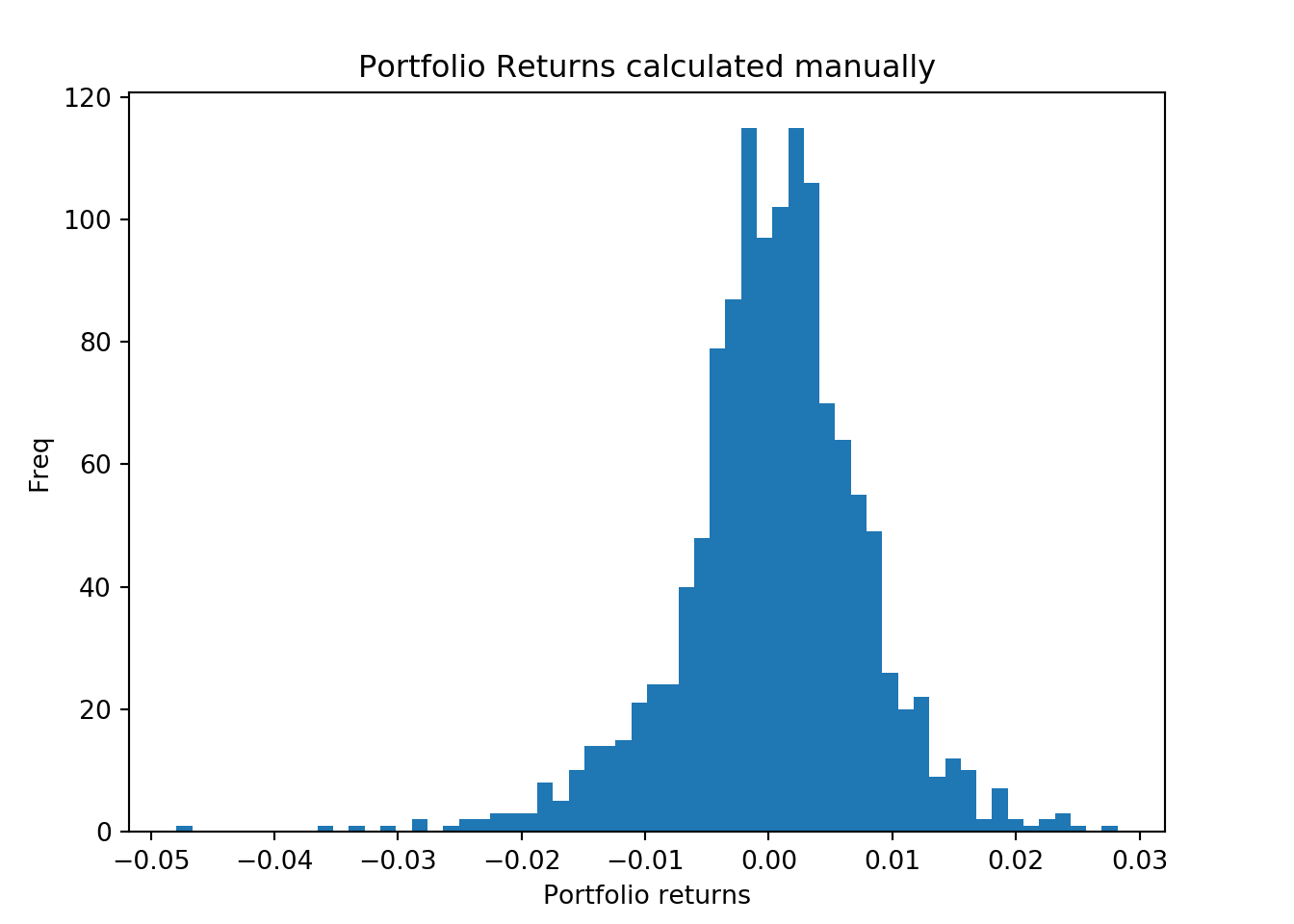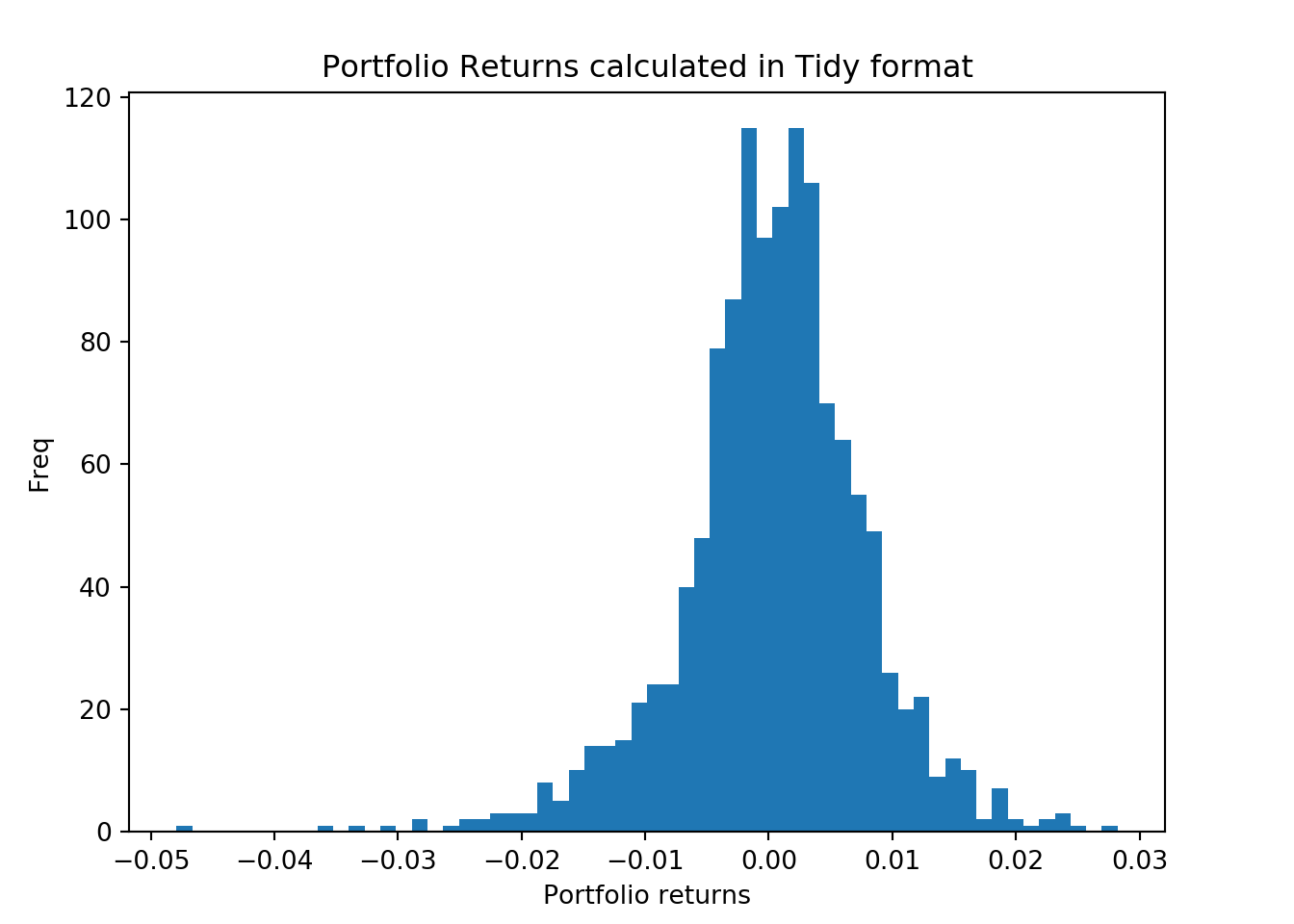## Calculating portfolio returns in Python

In this post we will learn to calculate the portfolio returns in Python. Since we are not aware of any modules that perform such calculations we will perform this calculation manually.

### Calculating portfolio returns using the formula

A portfolio return is the weighted average of individual assets in the portfolio.

Here is what we need

• Asset symbols that make up our portfolio
• Price data for the assets
• weights of assets
• Calculating the weighted average of our assets returns
• Adding them to get the portfolio returns

Lets first load the modules

``````import pandas as pd
import numpy as np
import matplotlib.pyplot as plt
import pandas_datareader as web``````

We will invest in the following assets

• Aggregate Bonds ETF (BND) 10%
• Small Cap ETF (VB) 20%
• Developed markets ETF (VEA) 25%
• S&P 500 ETF (VOO) 25%
• Emerging Markets ETF (VWO) 20%

So lets assign our assets to the symbols variable.

``symbols = ['VOO','VEA', 'VB', 'VWO','BND']``

Next we download the price data for the assets.

``````price_data = web.get_data_yahoo(symbols,
start = '2013-01-01',
end = '2018-03-01')``````

Now that we have the price data lets look at the head of this data.

``print(price_data.head())``
``````## Attributes       High               ...       Adj Close
## Symbols           BND         VB    ...             VOO        VWO
## Date                                ...
## 2013-01-02  83.940002  83.209999    ...      117.851250  38.347870
## 2013-01-03  83.930000  83.690002    ...      117.745369  38.120152
## 2013-01-04  83.779999  83.919998    ...      118.239380  38.187622
## 2013-01-07  83.760002  83.639999    ...      117.921799  37.858707
## 2013-01-08  83.849998  83.610001    ...      117.568985  37.546669
##
## [5 rows x 30 columns]``````

But we just need the Adjusted Closing price for our returns calculations. So lets select that columns.

``````price_data = price_data['Adj Close']
``````## Symbols           BND         VB        VEA         VOO        VWO
## Date
## 2013-01-02  71.491119  75.985794  29.420095  117.851250  38.347870
## 2013-01-03  71.278122  75.940125  29.124334  117.745369  38.120152
## 2013-01-04  71.388893  76.515572  29.288649  118.239380  38.187622
## 2013-01-07  71.337738  76.287193  29.140768  117.921799  37.858707
## 2013-01-08  71.405899  76.141068  28.984674  117.568985  37.546669``````

We can see that pandas has sorted our columns alphabetically so we need to align our weights correctly to the column names.

``w = [0.1,0.2,0.25,0.25,0.2]``

A quick check to see if our weights add to one.

``print(sum(w))``
``## 1.0``

Now we will calculate the asset returns in our portfolio.

``````ret_data = price_data.pct_change()[1:]
``````## Symbols          BND        VB       VEA       VOO       VWO
## Date
## 2013-01-03 -0.002979 -0.000601 -0.010053 -0.000898 -0.005938
## 2013-01-04  0.001554  0.007578  0.005642  0.004196  0.001770
## 2013-01-07 -0.000717 -0.002985 -0.005049 -0.002686 -0.008613
## 2013-01-08  0.000955 -0.001915 -0.005357 -0.002992 -0.008242
## 2013-01-09 -0.000358  0.004319  0.004818  0.003001  0.005840``````

Next we can calculate the weighted returns of our assets.

``````weighted_returns = (w * ret_data)
``````## Symbols          BND        VB       VEA       VOO       VWO
## Date
## 2013-01-03 -0.000298 -0.000120 -0.002513 -0.000225 -0.001188
## 2013-01-04  0.000155  0.001516  0.001410  0.001049  0.000354
## 2013-01-07 -0.000072 -0.000597 -0.001262 -0.000671 -0.001723
## 2013-01-08  0.000096 -0.000383 -0.001339 -0.000748 -0.001648
## 2013-01-09 -0.000036  0.000864  0.001205  0.000750  0.001168``````

Next the portfolio returns are simply the sum of the weighted returns of the assets. So lets add the rows.

``````port_ret = weighted_returns.sum(axis=1)
# axis =1 tells pandas we want to add
# the rows``````

Lets plot the histogram of the returns.

``````fig = plt.figure()
ax1.hist(port_ret, bins = 60)
ax1.set_xlabel('Portfolio returns')
ax1.set_ylabel("Freq")
ax1.set_title("Portfolio Returns calculated manually")
plt.show(); ``````## Tidy method in Python

In the example given in the R post we calculated the portfolio returns using the tidy dataframe. We can also calculate the returns using a tidy method in Python. To do that we need to reshape our returns dataframe and create a new weights table. We will then join the two and calculate the portfolio returns.

First lets create a weights table.

``````wts_table = pd.DataFrame({'symbol':symbols,
'wts':[0.25,0.25,0.2,0.2,0.1]})
``````##   symbol   wts
## 0    VOO  0.25
## 1    VEA  0.25
## 2     VB  0.20
## 3    VWO  0.20
## 4    BND  0.10``````

Next we will transform our returns data into a tidy data. First we need to reset the index and make the Date index into a separate column.

``````ret_data_tidy = pd.melt(ret_data.reset_index(),
id_vars='Date',
var_name='symbol',
value_name='ret')

``````##         Date symbol       ret
## 0 2013-01-03    BND -0.002979
## 1 2013-01-04    BND  0.001554
## 2 2013-01-07    BND -0.000717
## 3 2013-01-08    BND  0.000955
## 4 2013-01-09    BND -0.000358``````

We can see that the assets have been stacked on top of one another and Date has a separate column. Next we can merge our data by symbols.

``````ret_data_tidy_wts = pd.merge(ret_data_tidy,wts_table,on="symbol")
``````##         Date symbol       ret  wts
## 0 2013-01-03    BND -0.002979  0.1
## 1 2013-01-04    BND  0.001554  0.1
## 2 2013-01-07    BND -0.000717  0.1
## 3 2013-01-08    BND  0.000955  0.1
## 4 2013-01-09    BND -0.000358  0.1``````

We have the data in the desired form and now we can multiply our columns to find out the weighted average.

``````ret_data_tidy_wts['wt_returns'] = ret_data_tidy_wts['ret'] * ret_data_tidy_wts['wts']
``````##         Date symbol       ret  wts  wt_returns
## 0 2013-01-03    BND -0.002979  0.1   -0.000298
## 1 2013-01-04    BND  0.001554  0.1    0.000155
## 2 2013-01-07    BND -0.000717  0.1   -0.000072
## 3 2013-01-08    BND  0.000955  0.1    0.000096
## 4 2013-01-09    BND -0.000358  0.1   -0.000036``````

Finally we need to group our dataframe by date to calculate the daily returns on our portfolio.

``````port_ret_tidy = ret_data_tidy_wts.groupby("Date").sum()['wt_returns']
``````## Date
## 2013-01-03   -0.004344
## 2013-01-04    0.004484
## 2013-01-07   -0.004325
## 2013-01-08   -0.004023
## 2013-01-09    0.003951
## Name: wt_returns, dtype: float64``````

Now have the portfolio returns calculated in tidy format as well. We like this way since we can see which columns are getting multiplied. We can see that the results are same since the difference between the two is 0.

``````diff = port_ret - port_ret_tidy
print(diff.unique())``````
``## [0.]``

We can also plot the two histograms.

``````fig = plt.figure()
ax1.hist(port_ret, bins = 60) # manually calculated returns
ax1.set_xlabel('Portfolio returns')
ax1.set_ylabel("Freq")
ax1.set_title("Portfolio Returns calculated manually")
plt.show();````````````fig = plt.figure()
ax1.hist(port_ret_tidy, bins = 60) # Tidy returns
ax1.set_xlabel('Portfolio returns')
ax1.set_ylabel("Freq")
ax1.set_title("Portfolio Returns calculated in Tidy format")
plt.show();``````### Portfolio mean and standard deviation

Next we will calculate the portfolio mean and standard deviation, this is simple with the pandas module.

``````mean_ret = port_ret.mean()
std_returns = port_ret.std()
print(mean_ret)``````
``## 0.0003768744769855518``
``print(std_returns)``
``## 0.007587147407342516``

### Summary

In this post we learned

• To download asset prices in Python
• To calculate portfolio returns
• To transform the data into tidy format and calculate the returns